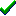# fg_3Daxisangle()Win32DirectXFastgraph/Light

Prototype

int fg_3Daxisangle (double x, double y, double z, int angle);

Function fg_3Daxisangle (ByVal x As Double, ByVal y As Double, ByVal z As Double, ByVal angle As Long) As Long

function fg_3Daxisangle (x, y, z : double; angle : integer) : integer;

Description

The fg_3Daxisangle() function rotates the viewer's orientation by the specified angle around an arbitrary axis through the 3D world space origin.

Parameters

x is the x coordinate of a vector defining the axis of rotation.

y is the y coordinate of a vector defining the axis of rotation.

z is the z coordinate of a vector defining the axis of rotation.

angle is the rotation angle, expressed in tenths of degrees. If angle is positive, the viewer rotates clockwise. If angle is negative, the viewer rotates counterclockwise.

Return value

If successful, fg_3Daxisangle() returns zero. It returns -1 if the vector's magnitude is too close to zero.

Restrictions

none

See also

Contents
Fastgraph Home Page

copyright 2001 Ted Gruber Software, Inc.